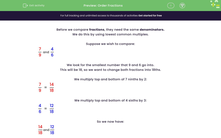# Use Common Denominators to Order Fractions

In this worksheet, students will use common denominators to place fractions in ascending order of size.Key stage:  KS 2

Curriculum topic:   Number: Fractions, Decimals and Percentages

Curriculum subtopic:   Compare and Order Fractions

Difficulty level:#### Worksheet Overview

Before we compare fractions, they need the same denominators.

We do this by using lowest common multiples.

Suppose we wish to compare:

 7 and 4 9 6

We look for the smallest number that 9 and 6 go into.

This will be 18, so we want to change both fractions into 18ths.

We multiply the numerator and the denominator of 7/9 by 2:

 7 = 14 9 18

We multiply the numerator and the denominator of 4/6 by 3:

 4 = 12 6 18

So we now have:

 14 and 12 18 18

 14 > 12 18 18

 7 > 4 9 6

Example

Place these fractions in ascending order of size.

 5 1 2 8 2 3

The lowest common multiple of 8, 2 and 3 is 24, because 3 × 8 = 24 and 12 × 2 = 24 and 8 × 3 = 24.

We multiply the numerator and the denominator of 5/8 by 3:

 5 = 15 8 24

We multiply the numerator and the denominator of 1/2 by 12:

 1 = 12 2 24

We multiply the numerator and the denominator of 2/3 by 8:

 2 = 16 3 24

 12 < 15 < 16 24 24 24

So the ascending order is:

 1 5 2 2 8 3

Let's have a go at some questions now.

### What is EdPlace?

We're your National Curriculum aligned online education content provider helping each child succeed in English, maths and science from year 1 to GCSE. With an EdPlace account you’ll be able to track and measure progress, helping each child achieve their best. We build confidence and attainment by personalising each child’s learning at a level that suits them.

Get started